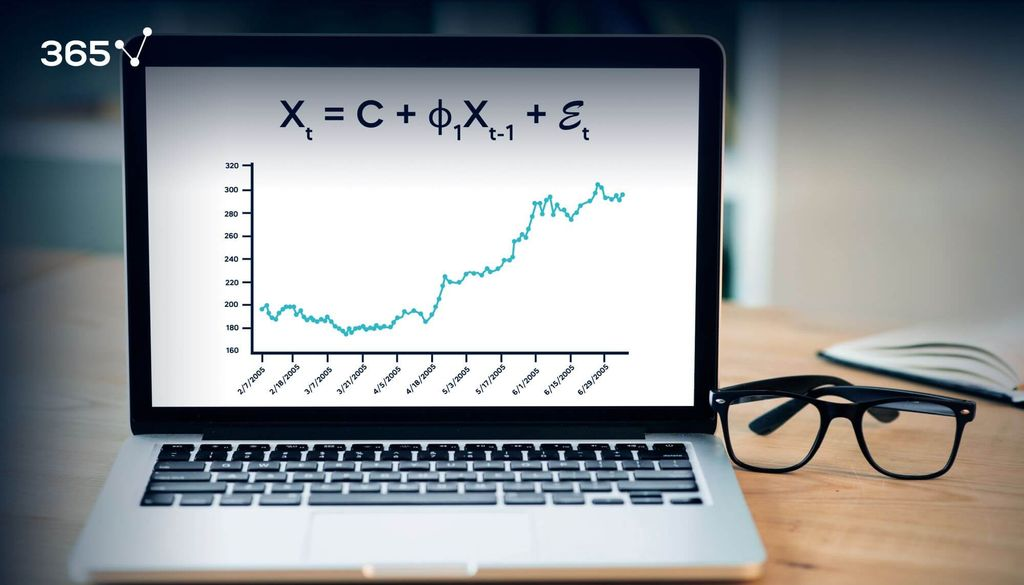# What Is an Autoregressive Model?In time-series, we often observe similarities between past and present values. That’s because we encounter autocorrelation within such data. In other words, by knowing the price of a product today, we can often make a rough prediction about its valuation tomorrow. So, in this tutorial, we’re going to discuss a model that reflects this correlation. – the autoregressive model.

## What is an Autoregressive Model?

The Autoregressive Model, or AR model for short, relies only on past period values to predict current ones. It’s a linear model, where current period values are a sum of past outcomes multiplied by a numeric factor. We denote it as AR(p), where “p” is called the order of the model and represents the number of lagged values we want to include.

For instance, if we take X as time-series variable, then an AR(1), also known as a simple autoregressive model, would look something like this:

Xt = C + ϕ1Xt-1 + ϵt

Let’s go over the different parts of this equation to make sure we understand the notion well.

### What is Xt-1?

For starters, Xt-1 represents the value of X during the previous period.

Let’s elaborate.

If “t” represents today and we have weekly values, then “t-1” represents last week. Hence, Xt-1 depicts the value recorded a week ago.

### What is ϕ1?

The coefficient ϕ1 is a numeric constant by which we multiply the lagged variable (Xt-1). You can interpret it as the part of the previous value which remains in the future. It’s good to note that these coefficients should always be between -1 and 1.

Let me explain why.

If the absolute value of the coefficient is greater than 1, then over time, it would blow up immeasurably.

This idea can seem confusing at first. So let’s take a look at a mathematical example.

Say, we have a time-series with 1000 observations and ϕ1 = 1.3 and C=0.

Then, X2 = 0 + 1.3 X1

Since X3 = 1.3 X2, we can substitute (1.3 X1) for X2 and get X3 = 1.3(1.3 X1) = 1.32 X1. Then, as the more periods accumulate (e.g. X50), the more the coefficient increases (1.349 X1).

When we get to the 1000th period, we would have X1000 = 1.3999 X1. This implies that the values keep on increasing and end up much higher than the initial one. This is obviously not a reliable way to predict the future.

### What is ϵt?

Okay, now the only part of the equation we need to break down is ϵt. It’s called the residual and represents the difference between our prediction for period t and the correct value (ϵt = yt - ŷt). These residuals are usually unpredictable differences because if there’s a pattern, it will be captured in the other incumbents of the model.

## How do we interpret the Autoregressive Model?

Now that we know what all the parts of the model represent, let’s try to interpret it. According to the equation, values at a given period (Xt) are equal to some portion (ϕ1) of values in the last period (Xt-1), plus some constant benchmark and unpredictable shocks ϵ t.

It is vital to understand that we don’t use just any autoregressive model on a given dataset. We first need to determine how many lags (past values) to include in our analysis.

## Autoregressive Model with More Lags

For example, a time-series about meteorological conditions wouldn’t solely rely on the weather statistics a day ago. It’s realistic to say it would use data from the last 7 days. Hence, the model should take into account values up to 7 periods back.

From a mathematical point of view, a model using two lags (AR(2)) would look as follows:

Xt = C + ϕ1 Xt-1 + ϕ2 Xt-2 + ϵt

As you can expect, a more complicated autoregressive model would consist of even more lagged values Xt-n, and their associated coefficients, ϕn . The more lags we include, the more complex our model becomes.

The more complicated the model, the more coefficients we have to determine and as a result, the more likely it is that some of them would not be significant.

Now, in general, a model that takes into account more data to make a prediction is usually better. However, if the coefficients (ϕ1, ϕ2,... ϕn) are not significantly different from 0, they would have no effect on the predicted values (since ϕk Xt-k = 0), so it makes little sense to include them in the model.

### Of course, determining the significance of these coefficients cannot be done by hand.

Lucky for us, Python is well-suited for the job. With convenient libraries like Pandas and Statsmodels, we can determine the best-fitting autoregressive model for any given data set.

If you want to learn more about implementing autoregressive models in Python, or how the model selection process works, make sure to check out our step-by-step Python tutorials.

If you’re new to Python, and you’re enthusiastic to learn more, this comprehensive article on learning Python programming will guide you all the way from the installation, through Python IDEs, Libraries, and frameworks, to the best Python career paths and job outlook.

## Ready to take the next step towards a career in data science?

Check out the complete Data Science Program today. Start with the fundamentals with our Statistics, Maths, and Excel courses, build up step-by-step experience with SQL, Python, R, and Tableau, and upgrade your skillset with Machine Learning, Deep Learning, Credit Risk Modeling, Time Series Analysis, and Customer Analytics in Python. If you still aren’t sure you want to turn your interest in data science into a solid career, we also offer a free preview version of the Data Science Program. You’ll receive 12 hours of beginner to advanced content for free. It’s a great way to see if the program is right for you.

#### Learn data science with industry expertsStart your career